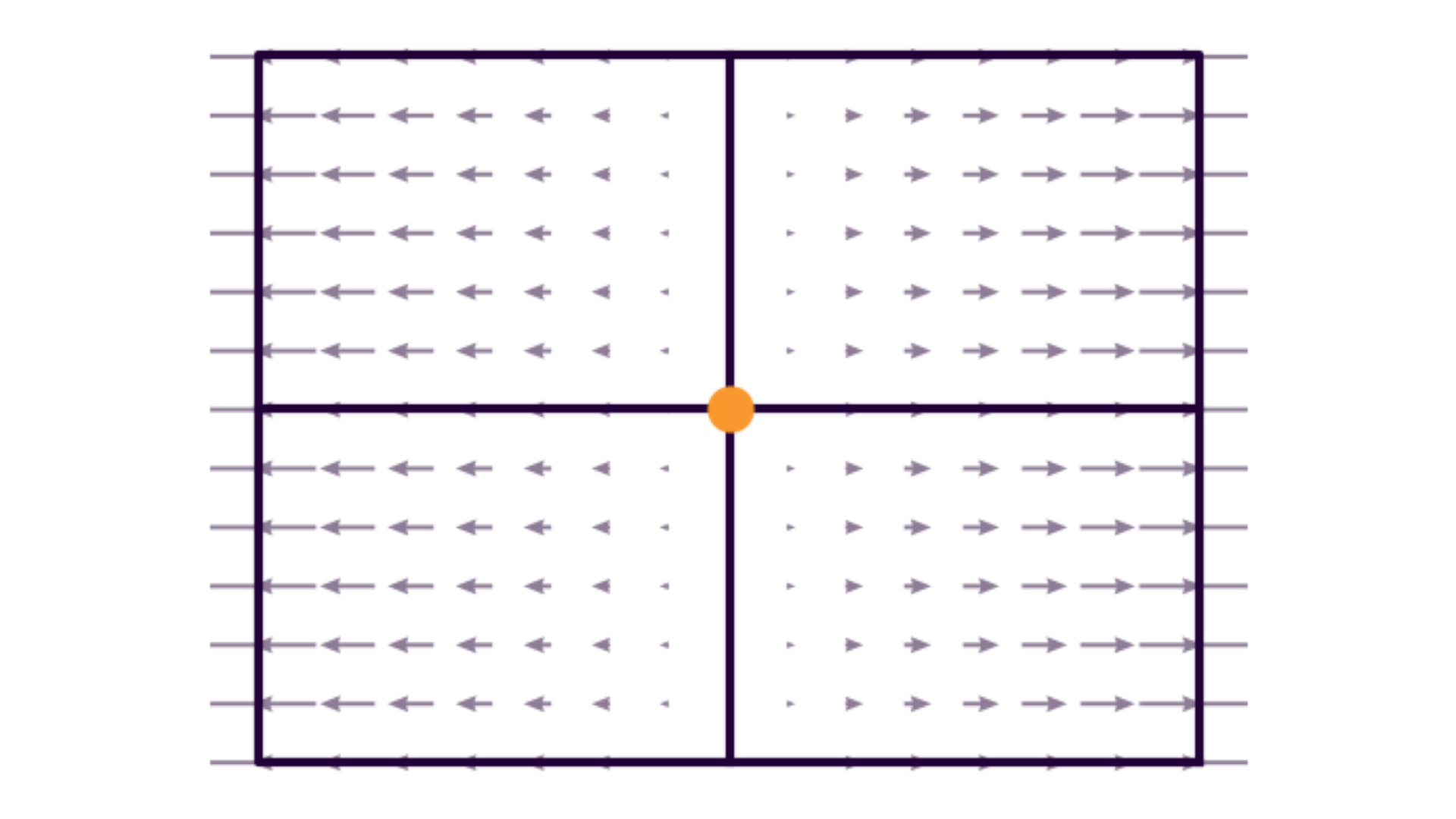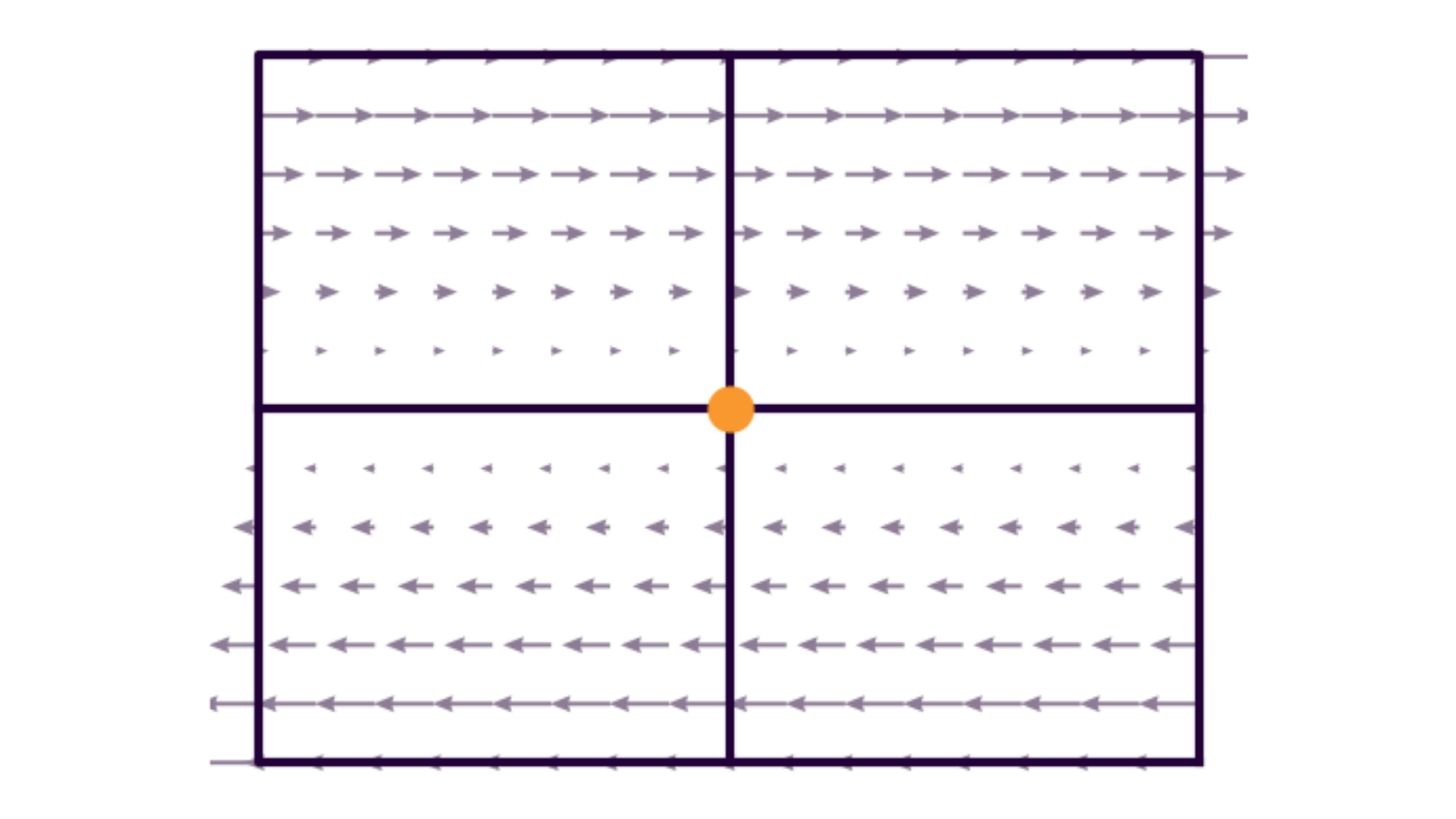# Affinity

## Characterization

The affinity model of our intrinsics aims to characterize errors or abberations in the image plane that appear as affine transformations of our image coordinates. These can be caused by various effects depending on the type of camera and underlying electronics that power the camera. Primarily, we categorize these into scale and shear effects.

Taking our generalized projection model, we can characterize these effects in the form of:

$\begin{bmatrix} x \\ y \end{bmatrix} = f \begin{bmatrix} X_c/ Z_c \\ Y_c / Z_c \end{bmatrix} + \begin{bmatrix} c_x \\ c_y \end{bmatrix} + g_{\mathsf{affinity}}(\bar{\textbf a})$

It is worth mentioning that while $$g_{\mathsf{affinity}}$$ can be modeled according to effects in $$x$$, effects in $$y$$, or both, the Tangram Vision Platform uses the convention of only applying our affinity model to the $$x$$ dimension for the sake of simplicity.

## Scale

The scale model aims to describe differences in pixel scaling in the $$x$$ (column) and $$y$$ (row) dimensions of our image plane.

In many perception systems, this is modeled as $$f_x$$ and $$f_y$$. See our explanation of the projection model for why Tangram doesn't follow this paradigm.

Scale effects may be due to:

1. Measured physical differences in pixel size (i.e. rectangular pixels). This is actually quite rare.
2. Differences between the clock frequencies of the analog CCD / CMOS clock and the sampling frequency of the Digital-Analog Converter (DAC) in the frame grabber.
3. Projective compensation effects resulting from an insufficient range of depths in the set of object space points used to calibrate. This is often the case if flat checkerboards are used for calibration.

The way that we model this effect is with a single linear coefficient $$a_1$$, as follows:

$g_{\mathsf{affinity}}(a_1) = \begin{bmatrix} a_1 (x - c_x) \\ 0 \end{bmatrix}$Read the Tangram Vision Blog post on focal length for a more complete explanation of these effects.

## Shear

Shear effects model a non-orthogonality between pixels. In effect, we would be modeling our image plane being shaped more like a rhombus than a square or rectangle.

For most full-frame cameras, this effect is not typically worth modeling, nor is it something that can be typically observed in practice. However, shear effects can be apparent in many line or push-broom cameras. This effect can be modeled as follows:

$g_{\mathsf{affinity}}(a_2) = \begin{bmatrix} a_2 (y - c_y) \\ 0 \end{bmatrix}$## Scale and Shear

Combining the two possible models above, we get a unified affinity model.

$g_{\mathsf{affinity}}(a_1, a_2) = \begin{bmatrix} a_1 (x - c_x) + a_2 (y - c_y) \\ 0 \end{bmatrix}$